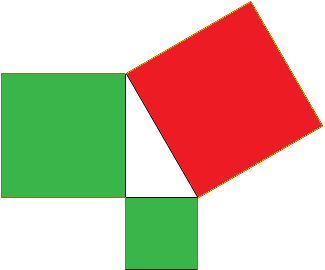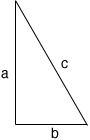Quandaries and Queries In triangle ABC. Angle ABC is 90 degrees. Side AB measures 34. Side BC measures 31. What does side AC measure? Bruce parent Secondary School difficulty Hi Bruce, This is an application of Pythagoras' Theorem which states For any right triangle the square on the hypotenuse is the sum of the squares on the other two sides. The hypotenuse is the side opposite the right angle and the statement means For any right triangle the area of the square on the hypotenuse is the sum of the areas of the squares on the other two sides. Hence in the diagram below the area of the red square is the sum of the areas of the green squares.Algebraically if the lengths of the sides of the triangle are a, b and c where c is the length of the hypotenuse then c2 = a2 + b2In the situation you describe a = 34 and b = 31 so c2 = 342 + 312 = 1156 + 961 = 2117 and hence c = sqrt(2117) = 46.01 to two decimal places. Penny Go to Math Central